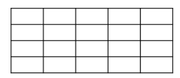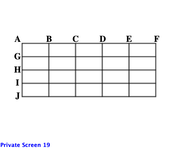• 7 CATs FREE!
If you earn 100 Forum Points

Engage in the Beat The GMAT forums to earn
100 points for $49 worth of Veritas practice GMATs FREEVERITAS PRACTICE GMAT EXAMS Earn 10 Points Per Post Earn 10 Points Per Thanks Earn 10 Points Per Upvote ## How many rectangles are found in the lattice below? ##### This topic has expert replies Elite Legendary Member Posts: 3656 Joined: 24 Jul 2015 Location: Las Vegas, USA Thanked: 19 times Followed by:36 members ### How many rectangles are found in the lattice below? by Max@Math Revolution » Mon Apr 08, 2019 11:07 pm ## Timer 00:00 ## Your Answer A B C D E ## Global Stats [GMAT math practice question] How many rectangles are found in the lattice below?A. 90 B. 100 C. 120 D. 150 E. 180Math Revolution Finish GMAT Quant Section with 10 minutes to spare. The one-and-only World's First Variable Approach for DS and IVY Approach for PS with ease, speed and accuracy. Only$149 for 3 month Online Course
Free Resources-30 day online access & Diagnostic Test
Email to : info@mathrevolution.com

GMAT Instructor
Posts: 15519
Joined: 25 May 2010
Location: New York, NY
Thanked: 13060 times
Followed by:1893 members
GMAT Score:790
by GMATGuruNY » Tue Apr 09, 2019 3:47 am
Max@Math Revolution wrote:[GMAT math practice question]

How many rectangles are found in the lattice below?A. 90
B. 100
C. 120
D. 150
E. 180To form a rectangle, we must combine as HORIZONTAL LENGTH with a VERTICAL LENGTH:

Horizontal length:
Number of ways to choose a horizontal length of 1:
AB, BC, CD, DE, EF = 5
Number of ways to choose a horizontal length of 2:
AC, BD, CE, DF = 4
Number of ways to choose a horizontal length of 3:
AC, BE, CF = 3
Number of ways to choose a horizontal length of 4:
Number of ways to choose a horizontal length of 5:
AF = 1
Total ways = 5+4+3+2+1 = 15

Vertical length:
Number of ways to choose a vertical length of 1:
AG, GH, HI, IJ = 4
Number of ways to choose a vertical length of 2:
AH, GI, HJ = 3
Number of ways to choose a vertical length of 3:
AI, GJ = 2
Number of ways to choose a vertical length of 4:
AJ = 1
Total ways = 4+3+2+1 = 10

To combine our horizontal options with our vertical options, we multiply:
15*10 = 150

Mitch Hunt
Private Tutor for the GMAT and GRE
GMATGuruNY@gmail.com

If you find one of my posts helpful, please take a moment to click on the "UPVOTE" icon.

Available for tutoring in NYC and long-distance.
Student Review #1
Student Review #2
Student Review #3

Elite Legendary Member
Posts: 3656
Joined: 24 Jul 2015
Location: Las Vegas, USA
Thanked: 19 times
Followed by:36 members
by Max@Math Revolution » Wed Apr 10, 2019 11:59 pm
=>

Each rectangle is uniquely determined by the intersections between two vertical lines and two horizontal lines.
Since we have 6 vertical lines and 5 horizontal lines, the number of rectangles is 6C2*5C2 = 15*10 = 150.